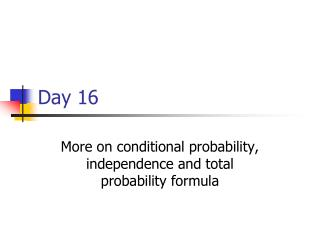DownloadDownload PresentationDay 16

# Day 16

Download Presentation## Day 16

- - - - - - - - - - - - - - - - - - - - - - - - - - - E N D - - - - - - - - - - - - - - - - - - - - - - - - - - -
##### Presentation Transcript

1. Day 16 More on conditional probability, independence and total probability formula

2. Conditional Probability and Independence • Example: • Roll a fair die twice, the first roll is 6 and the second roll is also 6. • Let A={first roll is 6} and B={second roll is 6} • we can see that since two rolls are independent, • P(A ∩ B)=P(A) P(B|A), but P(B|A)=P(B), therefore, • For independent events: • P(A ∩ B) =P(A)P(B)

3. Independence • Intuitively, if knowing the probability of one event, A, does not help us get the probability of the other event, B, we call the events A and B independent. • P(A|B)=P(A) (NOT P(B) !!!) • P(B|A)=P(B) • P(A∩B)=P(A)P(B)

4. Some more exercises on conditional probability • According to the survey on longevity, nowadays, 95% people will live past 20 years and 50% people will live past 65. • Tom just had his twentieth birthday on Sept 22nd, what is his chance of living past 65? • A={past 20} and B={past 65} • Here, we want P(B|A)=P(A∩B)/P(A) • Think about P(A∩B)…

5. More on conditional • Let A={ it will rain today }, B={ it will rain tomorrow }. We know that P(A)=0.4, P(B)=0.5 and P(A∩B)=0.3. Given that is rains today, what is the chance that it also rains tomorrow? (greater or less than P(B) ?) • Which probability are we looking for? • P(B|A) • P(B|A)=P(A∩B)/P(A)=0.3/0.4=0.75

6. Rain problem contd. • If it does not rain today, what is the chance that it rains tomorrow? • The probability we look for is? • P(B|Ac) • P(B|Ac)=P(Ac∩B)/P(Ac)

7. Some equalities • P(Ac∩B) + P(A∩B) = P(B) • P(Ac|B) + P(A|B) = 1

8. Keep pushing on the rain problem • Let A={ it rains on Saturday }, B={ I will go to Turkey Run state park}. I know that if it does not rain, I have a 80% chance going, if it rains, I may still go with 20% chance. If the chance of rain on Saturday is 60%, what is my total chance of going?

9. Total Probability Formula • If the outcome of an event, A, depends on the outcome of another event, B. The chance of A happening is: • P(A)=P(B1)P(A|B1)+ P(B2)P(A|B2)+…+ P(Bk)P(A|Bk), • B1, B2, …, Bk are the outcomes of B.

10. Example • Suppose you want to catch an early flight at Indy airport. You have the following plans: • 1. Let your friend drive you to the airport, with 20% chance of missing the flight. • 2. Taking the Lafayette Limo with a 25% chance of missing the flight. • 3. Hitch hike yourself with 50% of missing the flight. If your preference is 40% taking Lafayette Limo, 35% hitch hike and 25% asking your friend to drive, what is your chance of missing the flight.

11. Another Example • A product is manufactured over three separate machines: A, B and C. Machine A has 4% defective rate, machine B has 2% defective rate and machine C has 6% defective rate. If the percentages of products by machine are: 50%, 30% and 20%, what will be your defective rate?

12. A summary example • Toss a coin twice. For each toss P{H}=0.7 and P{T}=0.3. Find: • 1. P{two heads}; 2. P{two tails}

13. Summary example (contd) • 3. P{one head and one tail}

14. Yet another example • Someone is shooting at a target. If it is windy, he has a 40% chance hitting the target. If there is no wind, his chance is 70%. • A. If there is a 12% chance of being windy, what is his chance of hitting the target? • B. If he hits the target, what is the chance of it being windy???

15. Question B can only be solved using a new technique • Bayes theorem: • Bayes theorem deals with another type of question. • Think about conditional probability: it answers the question: if A happens then what is the chance for B to happen? ( A is a condition for B) • Bayes theorem answers another question: If B happens, what is the chance of A happening. (A is still a condition for B )

16. Formula • Think about total probability formula (two events case) • P(A)=P(B1)P(A|B1)+P(B2)P(A|B2) • Now we want to know P(B1|A)

17. Bayes’ Theorem • P(B1|A) = P(B1)P(A|B1) / [P(B1)P(A|B1)+P(B2)P(A|B2)] = P(B1)P(A|B1) / P(A) • P(B2|A) = P(B2)P(A|B2) / [P(B1)P(A|B1)+P(B2)P(A|B2)] = P(B2)P(A|B2) / P(A)